# TypeScript 循环

## TypeScript 循环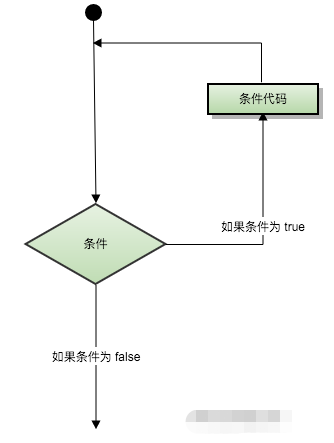## for 循环

TypeScript for循环用于多次执行一个语句序列，简化管理循环变量的代码。

### 语法

```for ( init; condition; increment ){
statement(s);
}```

for 循环的控制流程解析：

1. init 会首先被执行，且只会执行一次。这一步允许您声明并初始化任何循环控制变量。您也可以不在这里写任何语句，只要有一个分号出现即可。
2. 接下来，会判断 condition。如果为true，则执行循环主体。如果为false，则不执行循环主体，且控制流会跳转到紧接着for循环的下一条语句。
3. 在执行完for循环主体后，控制流会跳回上面的increment语句。该语句允许您更新循环控制变量。该语句可以留空，只要在条件后有一个分号出现即可。
4. 条件再次被判断。如果为true，则执行循环，这个过程会不断重复（循环主体，然后增加步值，再然后重新判断条件）。在条件变为false时，for循环终止。

condition 可以是任意的表达式，当条件为 true 时执行循环，当条件为 false 时，退出循环。

### 流程图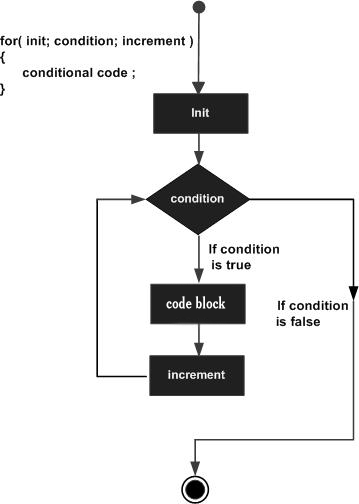### 实例

```//TypeScript代码
var num:number = 5;
var i:number;
var factorial = 1;

for(i = num;i>=1;i--) {
factorial *= i;
}
console.log(factorial)```

```//JavaScript 代码
var num = 5;
var num = 5;
var i;
var factorial = 1;
for (i = num; i >= 1; i--) {
factorial *= i;
}
console.log(factorial);
```

`120`

## for…in 循环

for…in 语句用于一组值的集合或列表进行迭代输出。

### 语法

```for (var val in list) {
//语句
}```

val需要为string或any类型。

### 实例

```//TypeScript代码
var j:any;
var n:any = "a b c"

for(j in n) {
console.log(n[j])
}```

```//JavaScript 代码
var num = 5;
var j;
var n = "a b c";
for (j in n) {
console.log(n[j]);
}
```

```a

b

c
```

## for…of、forEach、every和some循环

for…of语句创建一个循环来迭代可迭代的对象。在ES6中引入的for…of循环，以替代for…in和forEach()，并支持新的迭代协议。for…of允许你遍历Arrays（数组）,Strings（字符串）,Maps（映射）,Sets（集合）等可迭代的数据结构等。

```//TypeScript for...of 循环
let someArray = [1, "string", false];

for (let entry of someArray) {
console.log(entry); // 1, "string", false
}```

forEach、every 和 some 是 JavaScript 的循环语法，TypeScript 作为 JavaScript 的语法超集，当然默认也是支持的。

```//TypeScript forEach 循环
let list = [4, 5, 6];
list.forEach((val, idx, array) => {
// val: 当前值
// idx：当前index
// array: Array
});```
```//TypeScript every 循环
let list = [4, 5, 6];
list.every((val, idx, array) => {
// val: 当前值
// idx：当前index
// array: Array
return true; // Continues
// Return false will quit the iteration
});
```

## while 循环

while语句在给定条件为true时，重复执行语句或语句组。循环主体执行之前会先测试条件。

### 语法

```while(condition)
{
statement(s);
}```

condition 可以是任意的表达式，当条件为 true 时执行循环。 当条件为 false 时，程序流将退出循环。

### 流程图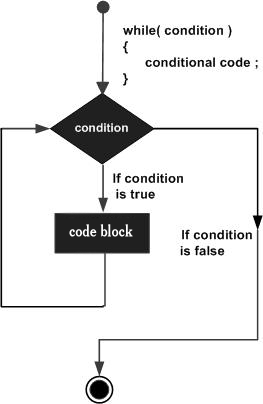### 实例

```//TypeScript代码
var num:number = 5;
var factorial:number = 1;

while(num >=1) {
factorial = factorial * num;
num--;
}
console.log("5 的阶乘为："+factorial);```

```//JavaScript 代码
var num = 5;
var num = 5;
var factorial = 1;
while (num >= 1) {
factorial = factorial * num;
num--;
}
console.log("5 的阶乘为：" + factorial);
```

`5 的阶乘为：120`

## do…while 循环

### 语法

```do
{
statement(s);
}while( condition );```

### 流程图### 实例

```//TypeScript代码
var n:number = 10;
do {
console.log(n);
n--;
} while(n>=0);```

```//JavaScript 代码
var num = 5;
var n = 10;
do {
console.log(n);
n--;
} while (n >= 0);```

```10
9
8
7
6
5
4
3
2
1
0```

## break 语句

break语句有以下两种用法：

1. 当 break 语句出现在一个循环内时，循环会立即终止，且程序流将继续执行紧接着循环的下一条语句。
2. 它可用于终止switch语句中的一个case。

`break;`

### 流程图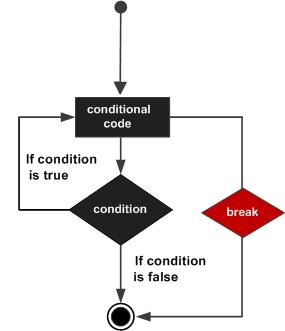### 实例

```//TypeScript
var i:number = 1
while(i<=10) {
if (i % 5 == 0) {
console.log ("在 1~10 之间第一个被 5 整除的数为 : "+i)
break     // 找到一个后退出循环
}
i++
}  // 输出 5 然后程序执行结束```

```//JavaScript
var i = 1;
while (i <= 10) {
if (i % 5 == 0) {
console.log("在 1~10 之间第一个被 5 整除的数为 : " + i);
break; // 找到一个后退出循环
}
i++;
} // 输出 5 然后程序执行结束```

`在 1~10 之间第一个被 5 整除的数为 : 5`

## continue 语句

continue语句有点像break语句。但它不是强制终止，continue会跳过当前循环中的代码，强迫开始下一次循环。

### 语法

`continue;`

### 流程图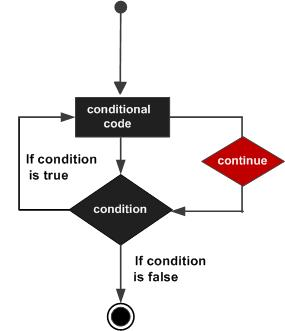### 实例

```//TypeScript
var num:number = 0
var count:number = 0;

for(num=0;num<=20;num++) {
if (num % 2==0) {
continue
}
count++
}
console.log ("0 ~20 之间的偶数个数为: "+count)    //输出10个偶数```

```//JavaScript
var num = 0;
var count = 0;
for (num = 0; num <= 20; num++) {
if (num % 2 == 0) {
continue;
}
count++;
}
console.log("0 ~20 之间的偶数个数为: " + count); //输出 10```

`0 ~20 之间的偶数个数为: 10`

## 无限循环

for 创建无限循环语法格式：

```for(;;) {
// 语句
}```

### 实例

```for(;;) {
console.log("这段代码会不停的执行")
}```

while 创建无限循环语法格式：

```while(true) {
// 语句
}```

### 实例

```while(true) {
console.log("这段代码会不停的执行")
}```

### 觉得文章有用就打赏一下文章作者

#### 支付宝扫一扫打赏#### 微信扫一扫打赏### 在线客服

•扫描二维码，微信联系